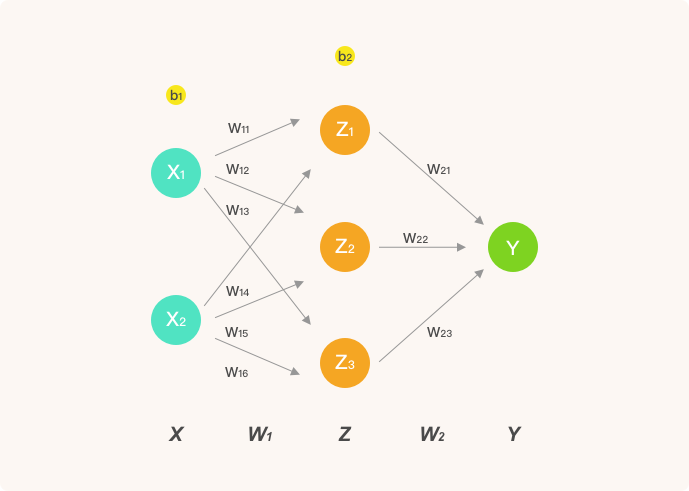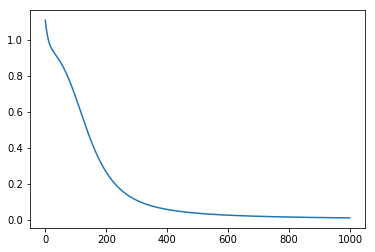## 人工神经网络数学推导及 Python 实现

1496 字 · 2028 阅读 · 2018 年 08 月 19 日### 定义神经网络结构$$\text {sigmoid} ( x ) = \frac { 1 } { 1 + e ^ { - x } }$$

$$\Delta sigmoid(x) = \operatorname { sigmoid } ( x ) ( 1 - \operatorname { sigmoid } ( x ) )$$

# sigmoid 函数
def sigmoid(x):
return 1 / (1 + np.exp(-x))

# sigmoid 函数求导
def sigmoid_derivative(x):
return sigmoid(x) * (1 - sigmoid(x))


### 前向传播

• $Z$ 表示隐含层输出， $Y$ 则为输出层最终输出。
• $w_{ij}$ 表示从第 $i$ 层的第 $j$ 个权重。

$$X = \left[ \begin{array} { l l } { x _ { 1 } } & { x _ { 2 } } \end{array} \right] \tag{2a}$$

$$W _ { 1 } = \left[ \begin{array} { l l l } { w _ { 11 } } & { w _ { 12 } } & { w _ { 13 } } \\ { w _ { 14 } } & { w _ { 15 } } & { w _ { 16 } } \end{array} \right] \tag{2b}$$

$$W _ { 2 } = \left[ \begin{array} { c } { w _ { 21 } } \\ { w _ { 22 } } \\ { w _ { 23 } } \end{array} \right] \tag{2c}$$

$$Z = \operatorname { sigmoid } \left( X \cdot W _ { 1 } \right) \tag{3a}$$

$$Y = \operatorname { sigmoid } \left( Z \cdot W _ { 2 } \right) \tag{3b}$$

### 反向传播

$$Y = \operatorname { sigmoid } \left( Z \cdot W _ { 2 } \right) \tag{4}$$

$$\frac { \partial \operatorname { Loss } ( y , Y ) } { \partial W _ { 2 } } = \frac { \partial \operatorname { Loss } ( y , Y ) } { \partial Y } \frac { \partial Y } { \partial W _ { 2 } } \tag{5a}$$

$$\frac { \partial \operatorname { Loss } ( y , Y ) } { \partial W _ { 2 } } = 2 ( Y - y ) * \Delta \operatorname { sigmoid } \left( Z \cdot W _ { 2 } \right) \cdot Z \tag{5b}$$

$$\frac { \partial \operatorname { Loss } ( y , Y ) } { \partial W _ { 1 } } = \frac { \partial \operatorname { Loss } ( y , Y ) } { \partial Y } \frac { \partial Y } { \partial Z } \frac { \partial Z } { \partial W _ { 1 } } \tag{6a}$$

$$\frac { \partial \operatorname { Loss } ( y , Y ) } { \partial W _ { 1 } } = 2 ( Y - y ) \Delta \operatorname { sigmoid } \left( Z \cdot W _ { 2 } \right) \cdot W _ { 2 } \Delta \operatorname { sigmoid } \left( X \cdot W _ { 1 } \right) \cdot X \tag{6b}$$

$$W _ { 1 } = W _ { 1 } + l r * \frac { \partial \operatorname { Loss } ( y , Y ) } { \partial W _ { 1 } } \tag{7a}$$

$$W _ { 2 } = W _ { 2 } + l r * \frac { \partial \operatorname { Loss } ( y , Y ) } { \partial W _ { 2 } } \tag{7b}$$

# 示例神经网络完整实现
class NeuralNetwork:

# 初始化参数
def __init__(self, X, y, lr):
self.input_layer = X
self.W1 = np.random.rand(self.input_layer.shape, 3) # 注意形状
self.W2 = np.random.rand(3, 1)
self.y = y
self.lr = lr
self.output_layer = np.zeros(self.y.shape)

# 前向传播
def forward(self):

# 实现公式 2，3
self.hidden_layer = sigmoid(np.dot(self.input_layer, self.W1))
self.output_layer = sigmoid(np.dot(self.hidden_layer, self.W2))

# 反向传播
def backward(self):

# 实现公式 5
d_W2 = np.dot(self.hidden_layer.T, (2 * (self.output_layer - self.y) *
sigmoid_derivative(np.dot(self.hidden_layer, self.W2))))

# 实现公式 6
d_W1 = np.dot(self.input_layer.T, (
np.dot(2 * (self.output_layer - self.y) * sigmoid_derivative(
np.dot(self.hidden_layer, self.W2)), self.W2.T) * sigmoid_derivative(
np.dot(self.input_layer, self.W1))))

# 参数更新，实现公式 7
# 因上方是 output_layer - y，此处为 -= 以保证符号一致
self.W1 -= self.lr * d_W1
self.W2 -= self.lr * d_W2


### 测试网络

import numpy as np
from matplotlib import pyplot as plt

# 测试数据
X = np.array([
[1, 0],
[0, 1],
[1, 0],
[1, 1],
])

y = np.array([, , , ])

nn = NeuralNetwork(X, y, lr=0.1) # 定义模型
loss_list = [] # 存放损失数值变化

for i in range(1000):
nn.forward() # 前向传播
nn.backward() # 反向传播
loss = np.sum((y - nn.output_layer) ** 2) # 计算平方损失
loss_list.append(loss)

print("final loss:", loss)
plt.plot(loss_list) # 绘制 loss 曲线变化图•